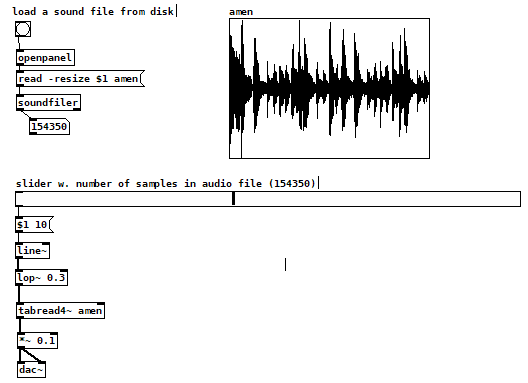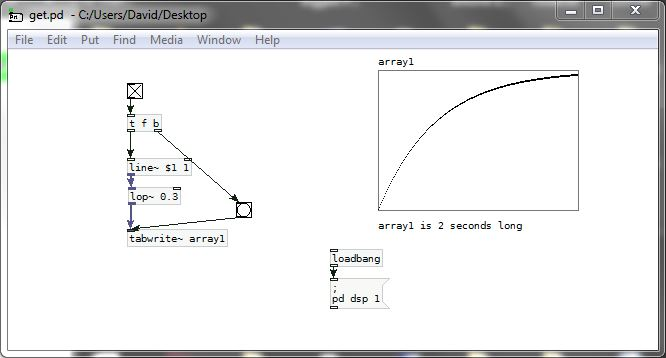• Explanation for lop~ object in this patch requested

Hi all,could someone be so kind as to explain the workings of the lop~ object in this patch to me?
This is a "simple" scrubbing bar that let's the user "scrub" through the loaded audio file by moving the horizontal slider. The lop~ was inserted to make this specific kind of "tape-start" / "tape-stop" effect that is familiar from some DAWs and DJing software.

It's hard for me to even formulate a question properly but it revolves about the fact that a filter object that usually is used for frequency-domain tasks (for example filtering high frequencies out from an audio stream) in this case is used as a time-domain-effect, i.e. creating a certain start-up / slow-down effect. I can't get my head around this. What's also confusing / interesting is that when you monitor the number outputs from the line~ and the lop~ with a snapshot~ object every 1ms the lop~ will output different numbers for some time while the line~ has already stopped outputting anything. How come? What does that mean for when you actually use lop~ as a "classic" audio filter to low-pass, say, a mic input or instrument recording.

I hope my question / confusion is somehow comprehensible. I am highly grateful for any explainations, preferably in layman's termsoyster

p.s.: the patch is from Andy Farell's super interesting lecture on Microsound - Granular Synthesis - Physical Modelling - Procedural Audio which I'm trying to follow along. youtube.com /watch?v=Qxqtc2bgDpw

• Posts 16 | Views 893
• @oystersauce High frequencies have fast changes between samples.... low frequencies slow.
So [lop~] allows slow changes in the signal domain.
get.pd
Fast changes are suppressed..... producing a curve....
But. The signal still has to get to the value requested. If its slope is being reduced then it will take more time....
In my patch above the time to change is 1 ms, so 1KHz, and the time is more than doubled with [lop~ 0.3] . I probably should have set [lop~ 3] to better match the curve in Andy Farnell's patch.

What does it mean for audio....? The phase is shifted to the right. There is a lag compared to the unfiltered signal..
David.• @whale-av thanks for your answer. I'm not quite there yet but I'll get there eventually• @whale-av Let me see if I understand this... in the theoretical part of Farnell's book, "Designing Sound" (7.3 Generating Digital Waveforms), he writes that an impulse spike contains all frequencies (based on the Uncertainty Principal). So when a sudden change in value is sent into the [lop~] (replacing the [line~] in your get.pd with a [sig~] doesn't change anything noticeably), it could be said that waves of all frequencies go from 0 to 1 or 1 to 0 at their respective speeds. By filtering out all the frequencies above 1, the fastest wave to make the transition becomes 1. For 0 to 1, this transition takes the shape of cos 18π-cos 24π (0.75-1) and for 1 to 0, cos 6π-cos 12π (0.25-5).

Am I on the right track? If so, what would be the equation to calculate the length of the transition based on a given filter pole a? I imagine it would involve dividing the wavelength/duration by 4 at some point, but I'm unsure of exactly how to do it.

• hi, the impulse response (the name for what happens in the time domain when you feed something an impulse, which has all "frequencies" of sine wave) of a causal low-pass filter generally has a "exponential" ramp characteristic in the time domain. This (of course) corresponds to the frequency response of a lowpass filter. (if you take the discrete fourier transform of the impulse response, it gives the frequency response).
if you lower the "frequency", which generally corresponds to less steepness/longer length of the exponential ramp in the time domain, then the fast changes in the signal will be "integrated" out more

see this thread for further info on using real poles in the time domain like this https://forum.puredata.info/topic/11356/signal-rate-separate-positive-negative-slew/4
also lag~ (which is like lop~ but you can set the coefficient directly) is now in my library, in case you are interested

• @s.elliot.perez also, here is an abstraction to convert time in milliseconds to go from 1 to .001 (60 db lower) to a coefficient for rpole~ or lag~ dbpole.zip

• @seb-harmonik-ar Thanks for the response, but I really wanted you to start with a "Yes" or "No" to my question "Am I on the right track?", lol. Since you talked about the impulse response as well, I take it to mean I was somewhat on the right track... but I was mistaken about the shape of the ramp being part of sine wave? Rather, it's just exponential for some reason? After that, you kind of lost me... using rpole~ instead of lop~ will just raise more questions for me, I'm afraid.

• @s.elliot.perez well, yes and noYou are right that the lower frequencies generally stick around and the higher ones get filtered out,
One thing to keep in mind is that a 1-pole filter has a very shallow frequency roll-off, so even if you set it at 1hz many other frequencies will "get through".
The exponential ramp is the linear combination of all of the sine waves (at their specific volumes) that the one-pole filter will let through at a specific "cutoff frequency", which is correlated to the steepness of the ramp. (A steep band-pass filter or even a steep low pass filter, on the other hand, might do what you say because it only lets through one narrow band of frequency)

• @s.elliot.perez I would recommend using lag~ if you are interested in using lop~ like this. it is exactly the same, but uses 60db time calculated from dbpole. There is a version of lag~ made in vanilla (lagtime~) in the other thread also.

Otherwise you would have to use the inverse of the function lop~ uses internally to pass in the right hz in order to get the specific coefficient that will give you the right 60db time, rather than passing in the coefficient directly.

• @s.elliot.perez lol actually I tried it it wasn't that bad, this should convert a 60db decay time in ms into a frequency for lop~ mstolop.pd
edit: basically you get the nth root of .001 (n = no. of samples) using ln & e^x and that's the pole value.

• @seb-harmonik.ar OK, I kind of get it. But what about [hip~] in this patch?

hipThing.pd

Whereas [lop~] goes from 0 to 1 when it gets a 1 and from 1 to 0 when receiving a 0, [hip~] goes from from 1 to 0 when it gets a 1 and -1 to 0 when it gets a 0. Why is this? I found this used to make a certain kind of impulse curve as can be seen to the right where the bang is. Slowing down the instant click to a 2ms change seems to create a mixture of both curves that goes from 1, past 0 and then back up to 0.

• @s.elliot.perez I don't think it has anything to do with [hip~]
I think with [vline~] things get complicated. It interpolates when it's time goes beyond 1 sample....... http://msp.ucsd.edu/techniques/v0.11/book-html/node52.html ..... but see the mention of fold-over as well in that page. That probably explains the -1 weirdness.
Using a time of 0.0227 ms at 44.1K pretty much gives the expected graph...... hipThing2.pd

[sig~]
An abrupt 1 or zero from [sig~]...... ?
I am not sure, but I can imagine that in this case the [hip~] sees a frequency of 0 Htz. It has no idea of the frequency because it receives a constant stream of incoming values of 0 or 1.

There used to be in "extended" in "extra/sigpack" an object called [impulse~] that generated single sample impulses at a defined frequency, and should be a better testing object. It depends on what you are looking for. The [vline~] timings above do work.
David.

• @s.elliot.perez if you think about it in the frequency domain, hip~ is supposed to "block" dc (constant values) so feeding it a stream on constant 1s will make it go to 0. (feeding constant anything will make it go to 0)

If we get into the design of this filter, as documented here http://msp.ucsd.edu/techniques/v0.11/book-html/node141.html#sect08.highpass we see that the highness filter is pretty much just a lowpass filter with a "zero" at 1. A "zero" at 1 has the effect of differentiating a signal, (whereas a "pole" at 1 would integrate it). so, when your signal goes from 1 to zero suddenly the derivative will be -1 for a split second, and then the "pole" will take over if the input stays constant (derivative of 0)
(& obviously the same thing happens when the signal jumps to 1, you get derivative of 1 that then ramps to 0)

• @seb-harmonik.ar When you say 'A "zero" at 1', what do you mean by 'at 1'? The constant stream of 1s from the [sig~] or [vline~]? Or are you saying that the 1 & 0 from [sig~] correspond to "pole" & "zero"?

• @s.elliot.perez a "zero" is a mathematical point in the complex plane where a function of a complex number is 0. the equation (1-az^-1) is 0 when z=a, we call "a" a zero of the function. so a zero at 1=a corresponds to the function 1-z^-1. this is the z-transform of a time domain equation y[n] = x[n] - x[n -1] where x is the input sequence, n is the current sample, and y is the output sequence. it is a "discrete" differentiator.
https://ccrma.stanford.edu/~jos/filters/

• @seb-harmonik.ar

@seb-harmonik.ar said:

the equation (1-az^-1) is 0 when z=a

Yes, I understand.

, we call "a" a zero of the function. so a zero at 1=a corresponds to the function 1-z^-1. this is the z-transform of a time domain equation y[n] = x[n] - x[n -1] where x is the input sequence, n is the current sample, and y is the output sequence. it is a "discrete" differentiator.

OK, so what sequence of input values is leading to the -1?

Posts 16 | Views 893
Internal error.

Oops! Looks like something went wrong!